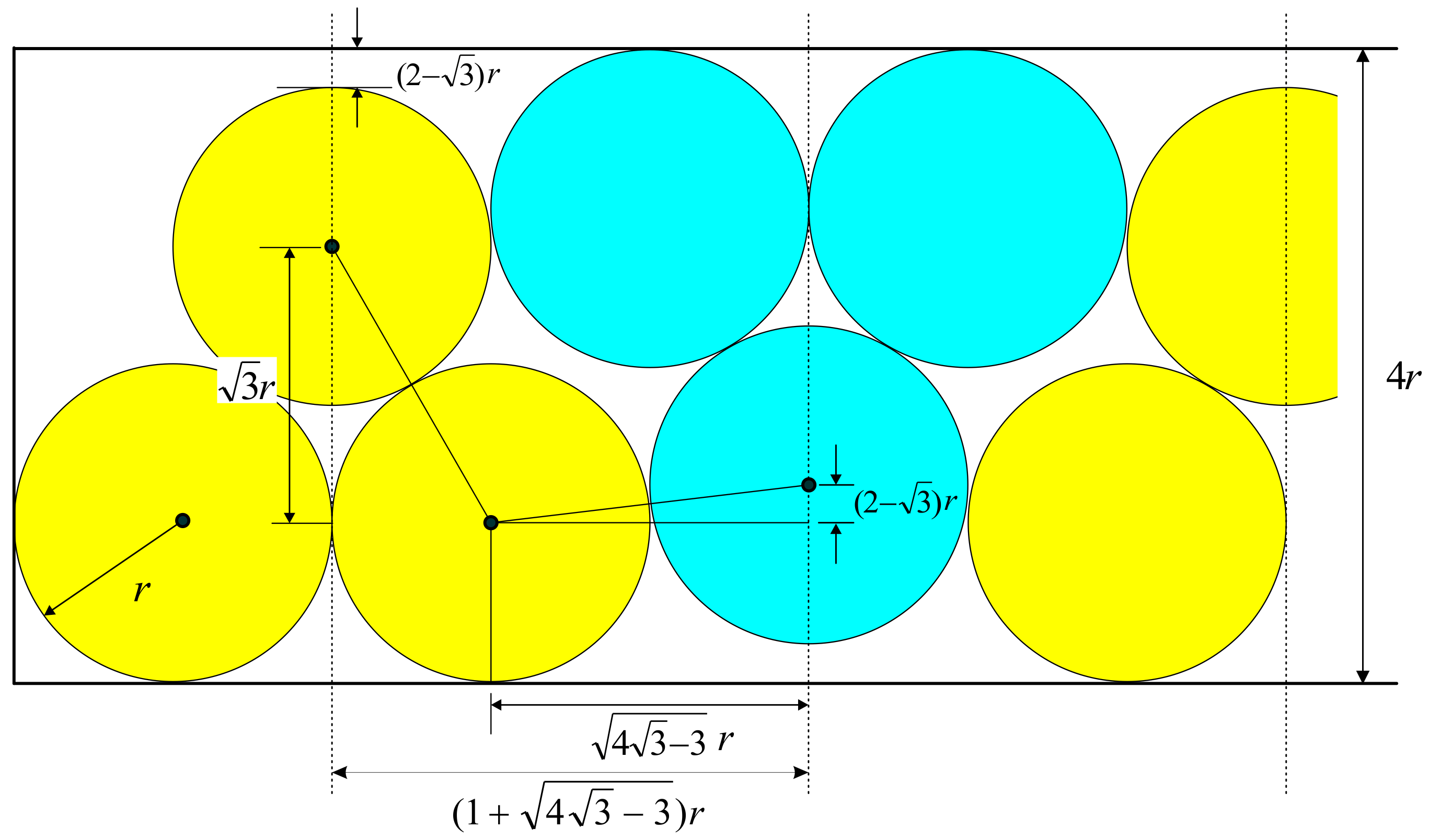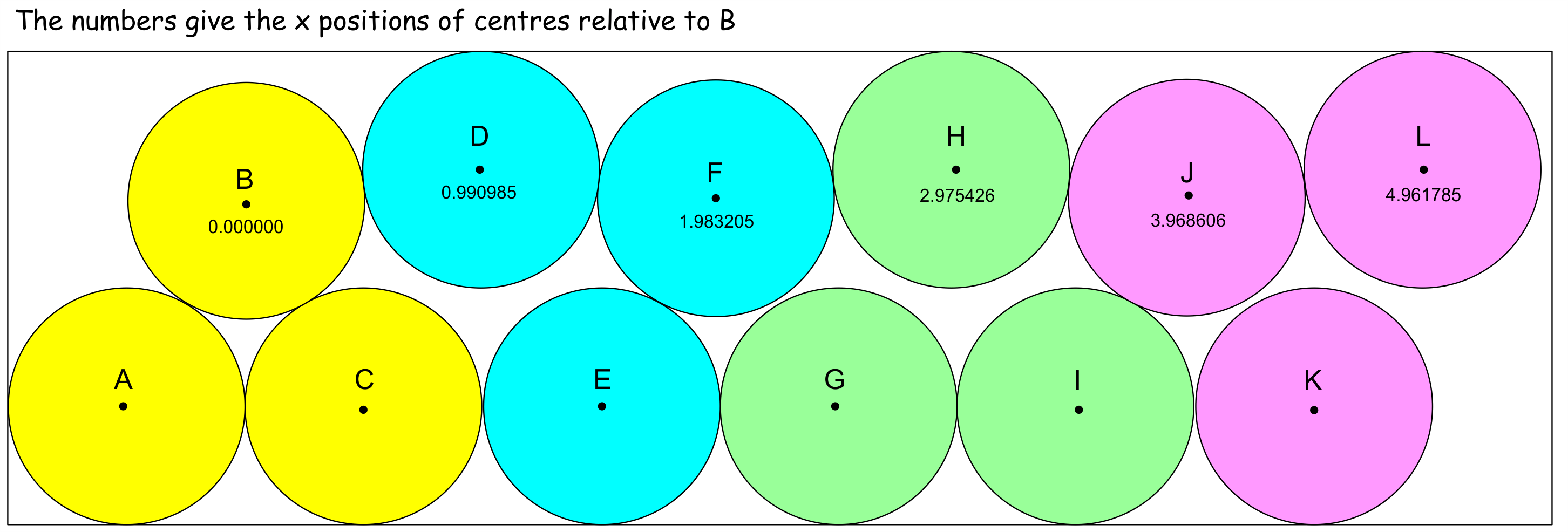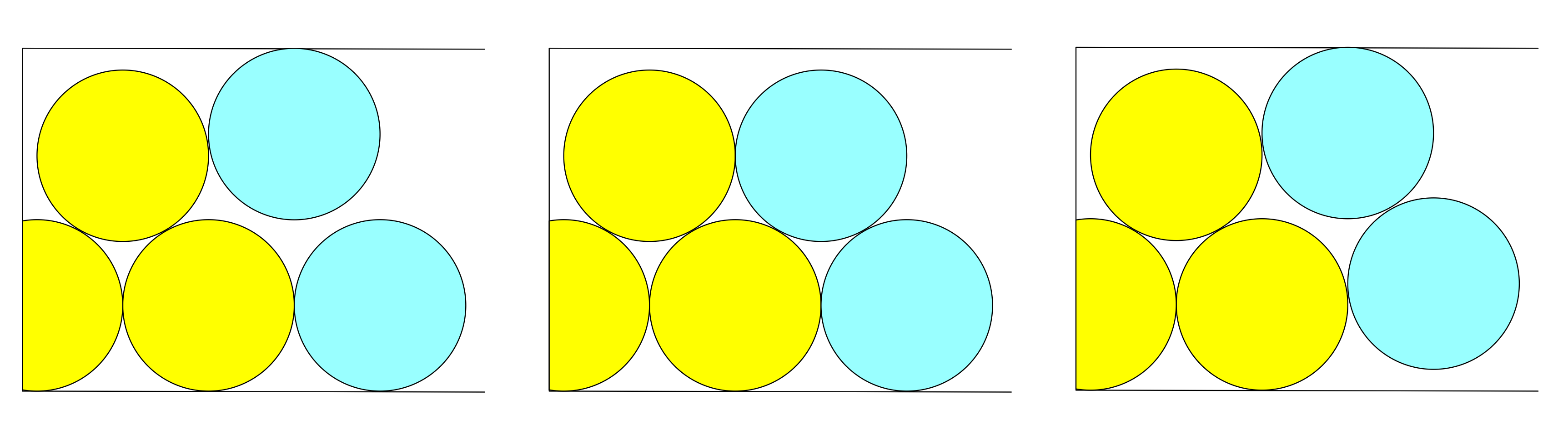# Radio Times Puzzle for Today No. 272

### by Professor Michael Paterson, University of Warwick

#### Published on Thursday 19 July 2018 (link)I have a tray of length 5 and width 2 so 10 round coins of width 1 will fit in it snugly without overlaps. No room for another. Similarly, a tray of length 50 will accommodate only 100 coins. Things get more interesting with a longer tray! A tray of length 500 and width 2 can accommodate at least 1001 coins. Show how this can be done. To be clear, this is just about fitting non-overlapping circles in rectangles, so no trickery with funny-shaped coins or thermal expansion coefficients! A useful hint is to start packing coins from the middle not from one end.

For a more mathematical challenge, what is the smallest $$n$$ so that $$2n+1$$ circles of diameter 1 can be packed without overlap in an $$n$$ by 2 rectangle? If you think you have a good answer to this, do let me know, but note that the really hard problem, still I think unsolved, is to prove the best optimum result.

John Owen (the coordinator for the Sunday Times Teasers) introduced this puzzle as a ‘bonus teaser’ on the Sunday Times Teasers Discussion Group in the comments on this page.   Subsequently he and others published possible solutions for the more difficult problem posed above, showing that a 2 by 166 tray holding 332 coins in a ‘2 by 2’ packing could accommodate 333 coins using a more compact packing using triples made up of three mutually touching coins.  This solution was, for example, described by Jim Randell on his Enigmatic Code site.

To the surprise of many, including me, Robert Brown then announced that he had found an improved solution that could fit 331 coins into a 2 by 165 tray and also thought that he could fit 329 coins into a 2 by 164 tray. He was, however, using measurements from scaled drawings and asked whether I could confirm this solutions using a more precise computer based approach. This launched my own study of the problem that I will now describe (I may refer to coins packed into trays or circles packed into rectangles in what follows).

The basis for the initial solution is illustrated here:Units of three mutually touching coins are placed as shown, alternately touching the lower and upper edges of the enclosing tray with small gaps of $$(2-\sqrt{3})r$$ between them and the opposite edge.    These triples are then placed repeatedly to fill the tray.  The distance between the centre-lines of adjacent triples (the dotted vertical lines) is given by $$(1 + \sqrt{4\sqrt{3} -3})r$$ which is approximately $$2.982r$$.   Except at each end, the two ‘half coins’ in each unit match with the half coins in adjacent (inverted) units to form full coins, which means that we can effectively fit three coins into a length of about $$2.982r$$.  The ‘packing ratio’ in the original  “2 by 2” packing is 2 coins in a length of $$2r$$ whereas our new packing achieves 3 coins in a length of about $$2.982r$$, albeit at the cost of needing an extra one half a coin width at each end. Despite this cost, however, as we add each of these new units we gain a small amount of space so that eventually we overcome the initial cost and then go on to provide enough space to add an additional coin. Using this method it proves possible to pack 333 coins into a 2 by 164 tray, one more than the regular “2 by 2” packing.

The way Robert Brown’s improved solutions work is to modify each end of the arrangement by adjusting the coin positions to achieve a more compact packing. An example of such an arrangement is shown here:In these ends, the triples are adjusted to align all lower coins on the lower edge of the tray, while the upper coins alternate between touching the upper edge and touching the two coins below them. These end arrangements can terminate on coins D, H, L … and can be applied at both ends of the overall sequence with regular triples being used in the middle section. A further possible adjustment is to raise coins G, K, … to touch the coins above them, a move which turns out to be slightly better in reducing the lengths of the ends.

Robert designed these coin layouts by hand but the approach can be automated. If we start from a triple and restrict ourselves to coins that touch tray edges or other coins, then there are three different positions where the next two coins can be placed as shown here:When we place another two coins, each of these three initial positions give another three positions for these coins giving 9 positions in total. Each time we repeat the process the number of coin positions triples so we end up with 3, 9, 27, … possibilities (in practice, the number is less because a lot of the positions are duplicates). If we now consider a specific coin in the sequences, the many different sequences reaching this coin will result in slightly different positions and we can pick the sequence that minimises its length and hence obtain the minimum length end for this number of coins. The resulting length measured from the centre of coin B to a tray edge that encloses the target ending coins is given here (with coins of unit radius):

$\begin{array}{|c|r|r|} \hline \text{Number of Coins} & \text{Lengh} \\ \hline 0 & 2.000000 \\ \hline 1 & 2.981970 \\ \hline 2 & 3.981970 \\ \hline 3 & 4.966411 \\ \hline 4 & 5.963939 & X \\ \hline 5 & 6.950852 \\ \hline 6 & 7.948380 & X \\ \hline 7 & 8.937211 \\ \hline 8 & 9.932821 & X \\ \hline 9 & 10.923484 \\ \hline 10 & 11.919137 & X \\ \hline 11 & 12.911202 & X \\ \hline 12 & 13.905453 \\ \hline 13 & 14.898920 \\ \hline 14 & 15.893171 & X \\ \hline 15 & 16.887423 & X \\ \hline 16 & 17.880890 & X \\ \hline \end{array}$

It can be seen from this table that $$n$$ coins can be fitted in a space that is slightly less than $$n+2$$ long. Note here that the yellow coins are counted as regular triples in the centre section, not in the end sections. But, to my surprise, minimising the lengths in this way does not find the shortest end sequences! This is because, in looking for minimum end lengths we have to consider ends built starting from both sides of a ‘2 + 2 half’ unit and sometimes the minimum length unit will be that constructed from the other end. Jim Randell used a neat way of working this out without by looking at each result and checking if a length with three less coins plus a ‘2+2 half’ unit was of shorter. This shows that the lengths marked with ‘X’s are not of minimum length and do not need to be used. So we are left with these possible end configurations:
$\begin{array}{|c|r|} \hline \text{Number of Coins} & \text{Lengh} \\ \hline 0 & 2.000000 \\ \hline 1 & 2.981970 \\ \hline 2 & 3.981970 \\ \hline 3 & 4.966411 \\ \hline 5 & 6.950852 \\ \hline 7 & 8.937211 \\ \hline 9 & 10.923484 \\ \hline 12 & 13.905453 \\ \hline 13 & 14.898920 \\ \hline \end{array}$

The method of looking for a fit is now as follows:

1. Select a target tray length and two possible end sequences
2. Subtract the lengths of the two end sequences from the tray length and divide the remainder by the length of of the triple unit
3. The integer part of the result is one less than the number of triple units needed to complete the packing
4. Add the numbers of coins in the centre section and the two ends and check if we have packed an extra coin

The Python program below automates this process and produces the following results for the smallest tray found that allows an extra coin (164). The lengths are given in coin widths and these solutions are the five that fit with the most free space left:

$\begin{array}{|c|c|r|r|} \hline \text{Ends} & \text{Triples} & \text{Length} & \text{Gap}\\ \hline (12, 12) & 102 & 163.997397 & 0.002603 \\ \hline (8, 10) & 104 & 163.997789 & 0.002211 \\ \hline (6, 12) & 104 & 163.998490 & 0.001510 \\ \hline (4, 8) & 106 & 163.999583 & 0.000416\\ \hline (6, 6) & 106 & 163.999583 & 0.000417 \\ \hline \end{array}$

Although we have used different methods, comparing these with those reported by Jim Randell on this page, there is complete agreement on these five solutions. I did at first believe that my length minimisation technique could find more solutions but Jim’s approach was better than mine and always found the same or better results. Here are the best results for the lengths 164, 165 and 166:

$\begin{array}{|c|c|c|r|r|r|} \hline \text{Tray Length} & \text{Ends} & \text{Triples} & \text{Coins} & \text{Length} & \text{Gap}\\ \hline 164 & (12, 12) & 102 & 329 & 163.997397 & 0.002603 \\ \hline 165 & (8, 12) & 104 & 331 & 164.991648 & 0.008352 \\ \hline 166 & (10, 12) & 104 & 333 & 165.985507 & 0.014493 \\ \hline \end{array}$